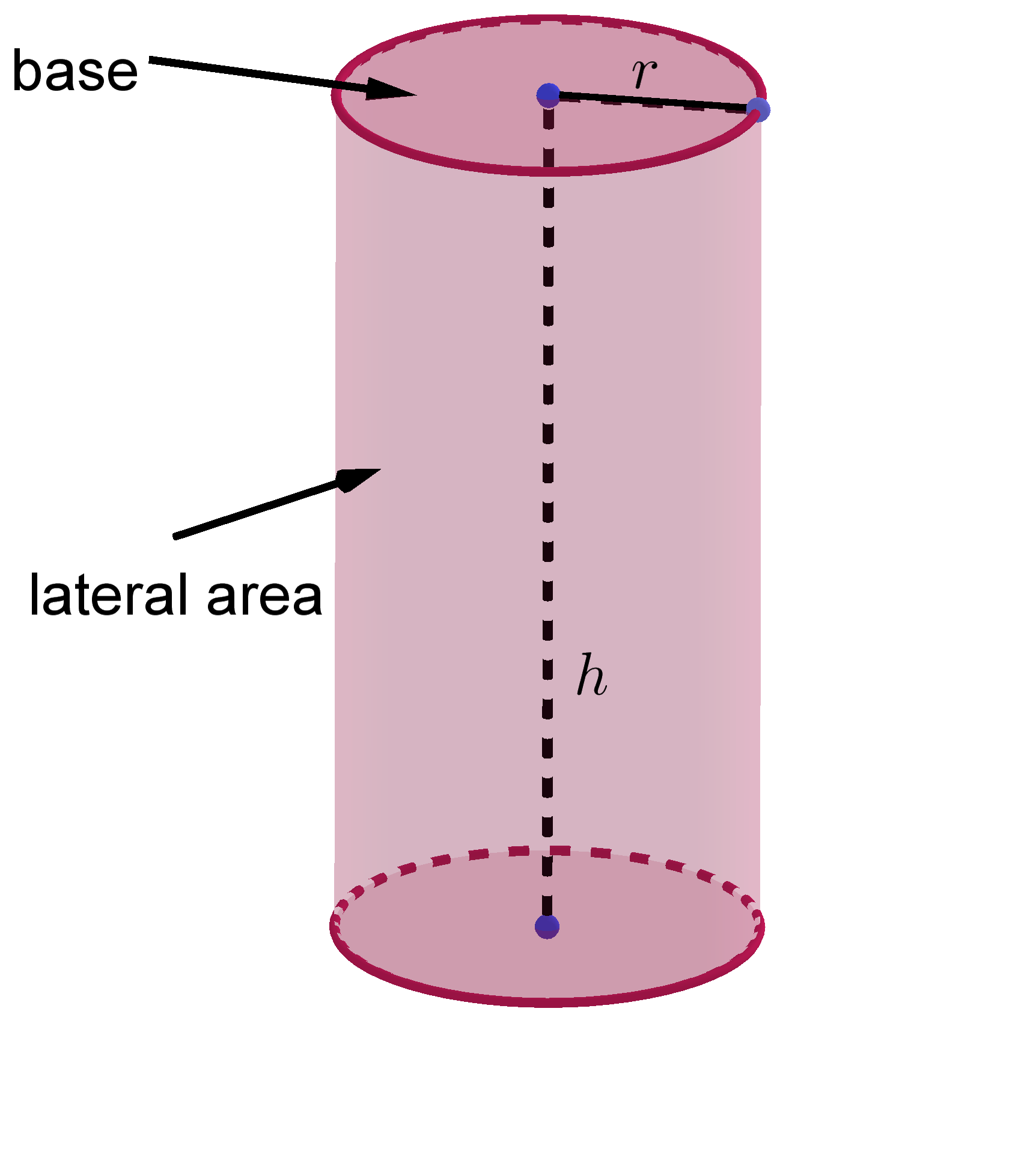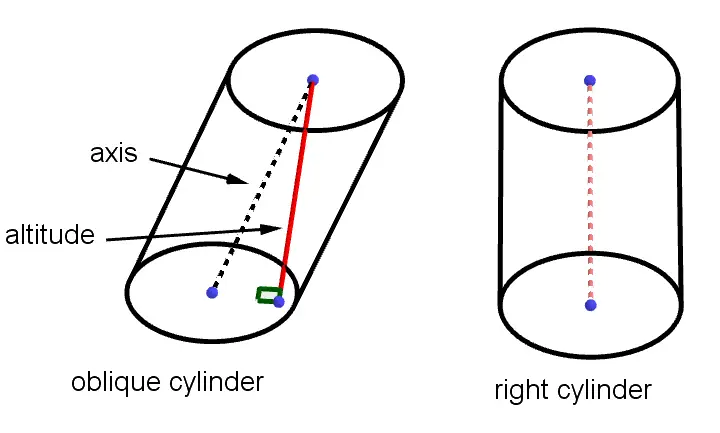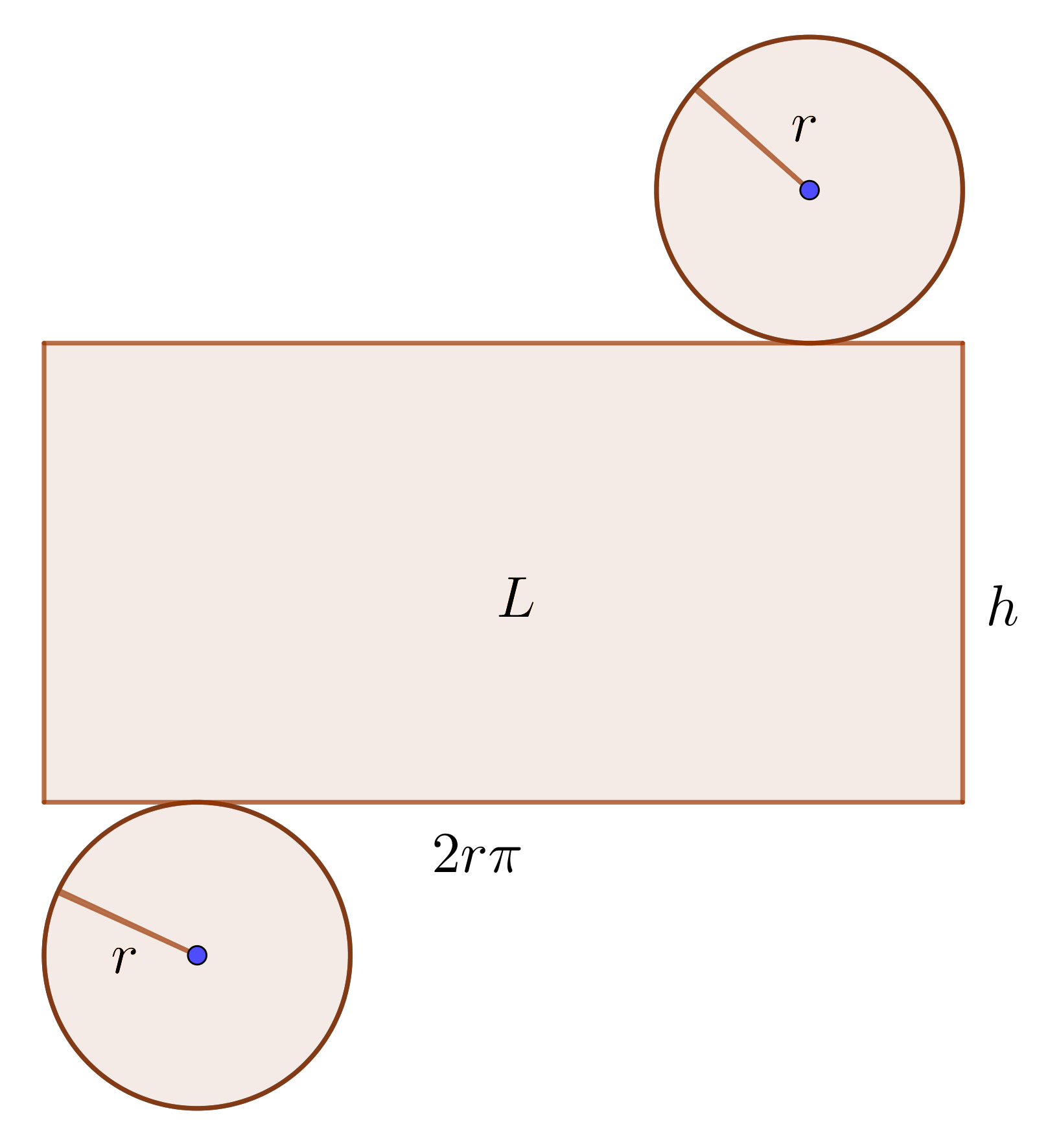# Volume & Surface Area of Cylinder Calculator

Radius
Height
Unit
Volume  =  785.3982in³<embed />
GENERATE WORK

## Cylinder Volume & Surface Area - work with steps

Input Data :
Radius = 5 in
Height = 10 in

Objective :
Find the volume of cylinder?

Formula :
Volume = πr2h

Solution :
Volume = 3.1416 x 52 x 10
= 3.1416 x 25 x 10
Volume = 785.3982 in³

Volume & surface area of cylinder calculator uses base radius length and height of a cylinder and calculates the surface area and volume of the cylinder. Cylinder calculator is an online Geometry tool requires base radius length and height of a cylinder. Using this calculator, we will understand methods of how to find the surface area and volume of the cylinder.
It is necessary to follow the next steps:

1. Enter the base radius length and height of a cylinder in the box. These values must be positive real numbers or parameter. Note that the length of a segment is always positive;
2. Press the "GENERATE WORK" button to make the computation;
3. Cylinder calculator will give the surface area and volume of a cylinder.
Input : Two positive real number or parameter as the base radius length and height of a cylinder;
Output : Two positive real numbers or variables as the surface area and volume of a cylinder and corresponding units after that.Cylinder Formula :
Surface Area of Cylinder Formula:
The surface area, $S$, of a cylinder is determined by the following formula $$S = 2\pi r(h+r)$$ where $r$ is the base radius length, $h$ is the height of a cylinder and $\pi\approx3.14$.

Volume of Cylinder Formula:
The volume, $V$, of a cylinder is determined by the following formula $$V = r^2\pi \times h$$ where $r$ is the base radius length, $h$ is the height of a cylinder and $\pi\approx3.14$.

## How to Find the Volume & Surface Area of a Cylinder?

A cylinder is a three-dimensional solid with congruent bases in a pair of parallel planes. These bases are congruent circles. The axis of the cylinder is the line segment with endpoints at centers of the bases. The height or altitude of a cylinder, denoted by $h$, is the perpendicular distance between its circular bases.There are two types of cylinder:

• Right Cylinder;
• Oblique Cylinder.
If the axis of cylinder is altitude, then the cylinder is right cylinder, otherwise the cylinder is oblique cylinder.If we cut a right cylinder and put it in a plane, we get a pattern or net for the cylinder. A net for the cylinder is shown below.The surface area of the cylinder is the sum of the areas of two congruent circles and a rectangle. The area of this rectangle is the lateral area of the cylinder. It is clear that the length of the rectangle is equal to the circumference of the base. Therefore, the lateral area of the cylinder is
$$L=2r\pi \times h$$
where $\pi\approx3.14$. Finally, to find the surface area of a cylinder, first find the lateral area and add the areas of base circles. The formula for the surface area of a right cylinder is
$$S=2r \pi h+2r^2 \pi=2 r \pi (h+r)$$
The volume of a cylinder is the measure of the amount of space that a cylinder contributes. The volume of a cylinder is the product of the area of the base and the height. Hence, $$V=B\times h=r^2\pi \times h$$ To find the volume of an oblique cylinder we will use the Cavalieri's Principle. Cavalieri's Principle states: If two solids have the same height and equal every cross-sectional area, then they have the same volume. If a cylinder has a base $B$ square and a height $h$, then its volume is $B\times h$, whether it is right or oblique.
The surface area of the cylinder is measured in units such as square centimeters $(cm^2)$, square meters $(m^2)$, square kilometers $(km^2)$, etc. The volume of a cylinder is measured in units such as cube centimeters $(cm^3)$, cube meters $(m^3)$, cube kilometers $(km^3)$ etc.

The cylinder volume & surface area work with steps shows the complete step-by-step calculation for finding the surface area and volume of the cylinder with the length of its base radius of $5\;in$ and height of $10\;in$ using the surface area and volume formulas. For any other values for the base radius lengths and height of a cylinder, just supply two positive real numbers and click on the Generate Work button. The grade school students may use this cylinder calculator to generate the work, verify the results of the surface area and volume of the three-dimensional bodies or do their homework problems efficiently.

### Real World Problems Using Cylinder Metrics

Calculating volume and surface area of cylinder play an important role in mathematics and real life as well. Formulas for volume & surface area of a cylinder can be used to find formulas for volume & surface area of the pipe. A knowledge of surface area formula is useful to calculate how much material we need to make some cylindrical shapes. The volume of cylinder formula is useful in determining the capacity of cylindrical shapes. For example, to design or find the capacity of a water tank, containers, bottle, cylindrical flasks, etc. With volume formula, we can better understand the density and capacity problems.

### Cylinder Practice Problems

Practice Problem 1:
Find the base radius length of a right cylinder if the surface area is $256$ square meters and the height is $18$ meters.

Practice Problem 2:
Silo has a cylindrical shape. Find the lateral area of a silo $20$ meters tall with the base radius length of $5$ meters. A second silo is $30$ meters tall. If both silos have the same lateral area, find the radius length of the second silo.

Practice Problem 3:
An Aquarium has cylindrical tanks. If the tank holds $100,000$ liters and is $5$ meter deep. Find the radius of the tank.

The cylinder calculator, formula, example calculation (work with steps), real world problems and practice problems would be very useful for grade school students (K-12 education) to understand the concept of volume & surface area of the cylinder. This concept can be of significance in geometry, to find the volume & surface area of cylinder and pipe. Real life problems on volume & surface area of the cylinder are very common, so this concept can be of great importance of solving problems.